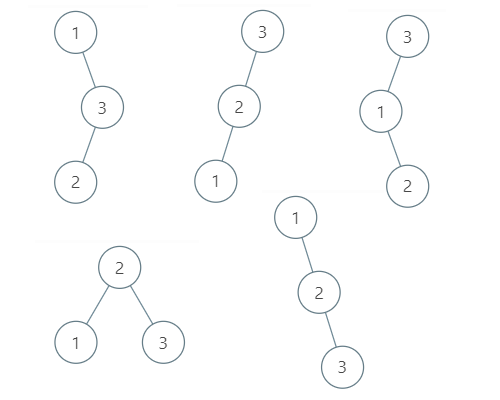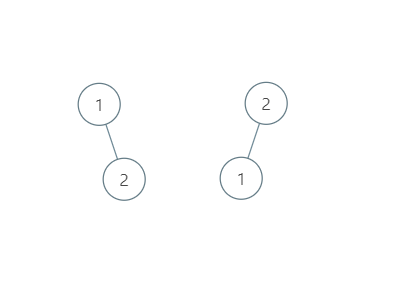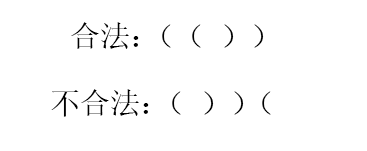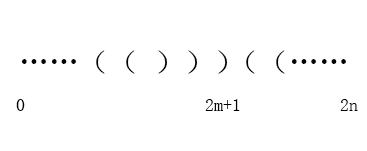# 【图解】从一道动态规划题带你领略『卡特兰数』是如何秒杀算法题的

### 一、 leetcode 96 号题

n = 3 时：### 二、动态规划1为根： 比 1 小的数只有 0，不用管。比 1 大的数有 2 和 3。

2为根： 比 2 小的是 1，比 2 大的是 3。这里只有 1 种。

3为根： 比 3 小的是 1 和 2，1 和 2 能生成多少种树呢？n = 2 的时候，按照我们之前的方法。

1 为根：比 1 大的数只有 2， 这里有 1 种。
2 为根：比 2 小的数只有 1， 这里有 1 种。

3

G(n) = $\displaystyle \sum^{n}_{i = 1}{G(i-1)}*G(n-i)$

d = 1; // 0 的时候特殊处理
for (int i = 1; i <= n; i++)
for (int j = 1; j <= i; j++)
d[i] += d[j-1] * d[i-j];


### 三、 Catalan公式

Catalan 递推项满足：

C(n) = C(0) * C(n-1) + C(1) * C(n-2) + … + C(n-2) * C(1) + C(n-1) * C(0)

Catalan 通项公式：$C_{n}$ = ${1}\over {n+1}$ $C_{2n}^{n}$

Catalan 递推公式1：$C_{n+1}$ = ${4n + 2}\over {n + 2}$ $C_{n}$

Catalan 性质：$C_{n}$ = $C_{2n}^{n}$ - $C_{2n}^{n-1}$

$C_{n}$ 的值，$C_{n+1}$ = ${4n + 2}\over {n + 2}$ $C_{n}$。公式顺推，代码中 n 次循环结束后的 ans 值就是 $C_{n}$ 对应的值。类比斐波那契最简解法。

	long ans = 1;
for(int i = 1; i <= n; i++)
ans = ans * 2 * (2 * i + 1) / (i + 2);
return (int) ans;


### 四、Catalan应用1. 首先计算一共的序列种数。n 对括号， 一共有 2n 个位置。我们选出其中 n 个位置放放置左括号，剩下的位置肯定就是右括号了。因此一共的种数为： $C_{2n} ^ {n}$
2. 接下来找出非法的括号序列数。每个非法的序列，在它的奇数位置，一定存在右括号数量大于左括号的数量。### 兄dei，如果觉得我写的不错，不妨帮个忙

1、关注我的原创微信公众号「帅地玩编程」，每天准时推送干货技术文章，专注于写算法 + 计算机基础知识（计算机网络+ 操作系统+数据库+Linux），听说关注了的不优秀也会变得优秀哦。

2、给俺点个赞呗，可以让更多的人看到这篇文章，顺便激励下我，嘻嘻。

### 作者简洁©️2019 CSDN 皮肤主题: 程序猿惹谁了 设计师: 上身试试